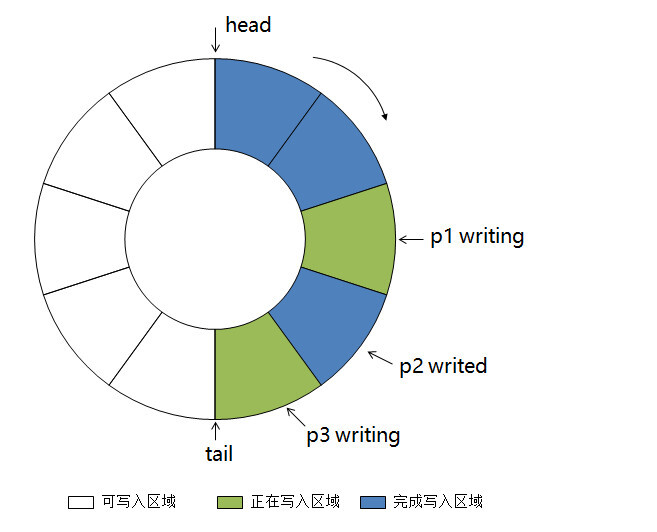手撕环形队列系列三：多生产者并行写入1. 生产者调用 ring_queue.request_push()方法，获得一个可以写入的 token；

2. 生产者通过 token 获取内存地址，向这个地址进行数据写入, 把数据都写好；

3. 生产者调用 token.finish()方法, 通知环形队列已经完成写入。// ring_queue.h#ifndef RING_QUEUE_H#define RING_QUEUE_H
typedef struct ring_queue_t { char* pbuf; char* pstate; int item_size; int capacity;
volatile int write_flag; volatile int read_flag;
volatile int head; volatile int tail; volatile int same_cycle;} ring_queue_t;
typedef struct ring_queue_token_t { ring_queue_t* pqueue; void* pitem; int idx;} ring_queue_token_t;
int ring_queue_init(ring_queue_t* pqueue, int item_size, int capacity);void ring_queue_destroy(ring_queue_t* pqueue);
int ring_queue_request_push(ring_queue_t* pqueue, ring_queue_token_t* ptoken);void* ring_queue_token_item(ring_queue_token_t* ptoken);int ring_queue_token_finish(ring_queue_token_t* ptoken);
int ring_queue_pop(ring_queue_t* pqueue, void* pitem);
int ring_queue_is_empty(ring_queue_t* pqueue);int ring_queue_is_full(ring_queue_t* pqueue);
#endif

// ring_queue.c#include "ring_queue.h"
#include <stdlib.h>#include <string.h>
#define CAS(ptr, old, new) __sync_bool_compare_and_swap(ptr, old, new)
enum ItemState { ITEM_BLANK, ITEM_WRITING, ITEM_WRITED,};
int ring_queue_init(ring_queue_t* pqueue, int item_size, int capacity) { memset(pqueue, 0, sizeof(*pqueue)); pqueue->pbuf = (char*)malloc(item_size * capacity); pqueue->pstate = (char*)malloc(capacity); if (!pqueue->pbuf || !pqueue->pstate) { return -1; }
pqueue->item_size = item_size; pqueue->capacity = capacity; pqueue->same_cycle = 1; memset(pqueue->pstate, ITEM_BLANK, capacity); return 0;}
void ring_queue_destroy(ring_queue_t* pqueue) { free(pqueue->pbuf); free(pqueue->pstate); memset(pqueue, 0, sizeof(*pqueue));}
int ring_queue_request_push(ring_queue_t* pqueue, ring_queue_token_t* ptoken) { // try to lock write flag while (1) { if (ring_queue_is_full(pqueue)) { return -1; }
if (CAS(&pqueue->write_flag, 0, 1)) { // set write flag successfully break; } }
// generate token int idx = pqueue->tail; pqueue->pstate[idx] = ITEM_WRITING; ptoken->pqueue = pqueue; ptoken->idx = idx; ptoken->pitem = pqueue->pbuf + (idx * pqueue->item_size); idx = (idx + 1) % pqueue->capacity; if (0 == idx) { // a new cycle pqueue->same_cycle = 0; // tail is not the same cycle with head } pqueue->tail = idx;
// unlock write flag CAS(&pqueue->write_flag, 1, 0);
return 0;}
void* ring_queue_token_item(ring_queue_token_t* ptoken) { return ptoken->pitem;}
int ring_queue_token_finish(ring_queue_token_t* ptoken) { // set item commit state ring_queue_t* pq = ptoken->pqueue; pq->pstate[ptoken->idx] = ITEM_WRITED;
return 0;}
int ring_queue_pop(ring_queue_t* pqueue, void* pitem) { // try to set read flag while (1) { if (ring_queue_is_empty(pqueue)) { return -1; }
if (CAS(&pqueue->read_flag, 0, 1)) { // set read flag successfully break; } }
int iret = -2; if (ITEM_WRITED == pqueue->pstate[pqueue->head]) { // read data memcpy(pitem, pqueue->pbuf + pqueue->head * pqueue->item_size, pqueue->item_size); pqueue->head = (pqueue->head + 1) % pqueue->capacity; if (0 == pqueue->head) { pqueue->same_cycle = 1; // head is now the same cycle with tail } iret = 0; // succ }
return iret;}

int ring_queue_is_empty(ring_queue_t* pqueue) { return (pqueue->head == pqueue->tail) && pqueue->same_cycle;}
int ring_queue_is_full(ring_queue_t* pqueue) { return (pqueue->head == pqueue->tail) && !pqueue->same_cycle;}

// test_ring_queue.c#include "ring_queue.h"#include <stdio.h>
static void test_push(ring_queue_t* pq, ring_queue_token_t* ptk, int val);static void test_pop(ring_queue_t* pq);
int main() { ring_queue_t queue, *pq = &queue; int iret = ring_queue_init(pq, sizeof(int), 3); iret = ring_queue_is_empty(pq); printf("ring_queue is%s empty!\n", iret ? "" : " not");
ring_queue_token_t tk1, tk2, tk3, tk4; iret = ring_queue_request_push(pq, &tk1); iret = ring_queue_request_push(pq, &tk2); iret = ring_queue_request_push(pq, &tk3); iret = ring_queue_request_push(pq, &tk4);
int val = 1; test_push(pq, &tk1, val++); test_push(pq, &tk2, val++); test_push(pq, &tk3, val++);
iret = ring_queue_is_full(pq); printf("ring_queue is%s full!\n", iret ? "" : " not");
test_pop(pq);
iret = ring_queue_request_push(pq, &tk4); test_push(pq, &tk4, val++);
test_pop(pq); test_pop(pq); test_pop(pq); test_pop(pq);
ring_queue_destroy(pq); return 0;}
static void test_push(ring_queue_t* pq, ring_queue_token_t* ptk, int val) { int* p = (int*)ring_queue_token_item(ptk); *p = val; int iret = ring_queue_token_finish(ptk); if (0 == iret) { printf("ring_queue_push succ, val = %d\n", val); } else { printf("ring_queue_push failed! iret = %d\n", iret); }}
static void test_pop(ring_queue_t* pq) { int val = -1; int iret = ring_queue_pop(pq, &val); if (0 == iret) { printf("ring_queue_pop succ, val = %d\n", val); } else { printf("ring_queue_pop failed! iret = %d\n", iret); }}

\$ ./test_ring_queuering_queue is empty!ring_queue_push succ, val = 1ring_queue_push succ, val = 2ring_queue_push succ, val = 3ring_queue is full!ring_queue_pop succ, val = 1ring_queue_push succ, val = 4ring_queue_pop succ, val = 2ring_queue_pop succ, val = 3ring_queue_pop succ, val = 4ring_queue_pop failed! iret = -1评论